Courses

Test: Counting In Groups - 1

10 Questions MCQ Test Mathematics for Class 2: NCERT | Test: Counting In Groups - 1

Description
Attempt Test: Counting In Groups - 1 | 10 questions in 20 minutes | Mock test for Class 2 preparation | Free important questions MCQ to study Mathematics for Class 2: NCERT for Class 2 Exam | Download free PDF with solutions
QUESTION: 1

Add the following number of cups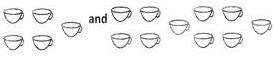Solution: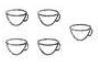= 5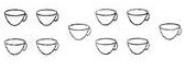= 10

So, we have 5 and 10 cups respecitively.

Hence,  adding these will give 5 + 10 = 15 cups

QUESTION: 2

Add the following: 45, 67 and 70.

Solution:

Firstly add two numbers = 45 + 67 = 112
Then, add remaining number = 112 + 70 = 182

QUESTION: 3

Fill in the blank from the options given below.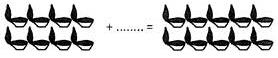Solution: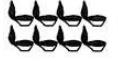In counting, number of diyas above are 8 in number.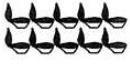In counting, number of diyas in second figure are 10 in number.

Hence, if we add two more diyas to the first figure we will get 10 diyas.

QUESTION: 4

76 + 54 + 89 + 32 = ?

Solution:

► (76 + 54) + (89 + 32)
► 130 + 121
► 251

QUESTION: 5

John bought 56 kg of wheat and 66 kg of rice. How much quantities of articles he bought in total?

Solution:

Wheat bought by John in Kg = 56
Rice bought by John in Kg = 66
In total he bought = 56 Kg + 66 Kg = 122 kg

QUESTION: 6

Which one of the following is correct about the addends of the given picture?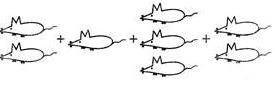Solution:

In picture given:
2 mice + 1 mice + 3 mice + 2 mice
so, 2 + 1 + 3 + 2 is the correct answer

QUESTION: 7

There are 25 people in an event. After some time 45 people join them. How many people are there in total, in the event?

Solution:

Number of people already present in the event = 25

Number of people joined the party after sometime = 45

Total number of people in the party = 25 + 45 = 70

QUESTION: 8

Find out the addition of the numbers given inside the circle.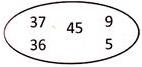Solution:

Let's take two numbers on the left side of the circle and add them = 37 + 36 = 73

Now take two number on the right side of the circle = 9 + 5 = 14

Let's add both the results we get = 73 + 14 = 87

Then add this number to the number in the middle of the circle= 87 + 45 = 132

Or,

► (37 + 36) + 45 + (9 + 5)
► 73 + 45 + 14
► 118 + 14
► 132

QUESTION: 9

Solution:

Rs. 60 + Rs. 56 + Rs. 29

= Rs. 116 + Rs. 29
= Rs. 145

QUESTION: 10

Cost of 2 text books is Rs.89 and 3 note books is Rs.67. How much money is paid in all if the cost of one drawing book is Rs.23?

Solution:

Cost of 2 text books = Rs. 89
Cost of 3 notebooks = Rs. 67
Cost of 1 drawing book = Rs. 23

Total cost = Rs. 89 + Rs. 67 + Rs. 23
= 179Use Code STAYHOME200 and get INR 200 additional OFF Use Coupon Code

Track your progress, build streaks, highlight & save important lessons and more!

Similar ContentRelated tests